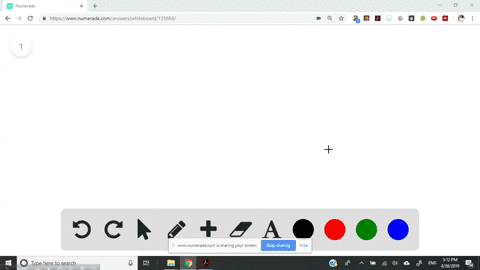Sign up for our free STEM online summer camps starting June 1st!View Summer Courses### The following diagram represents a high-temperatu…

03:55University of New Mexico

Need more help? Fill out this quick form to get professional live tutoring.

Get live tutoring
Problem 5

Glycine, an amino acid used by organisms to make proteins, is represented by the following molecular model.
(a) Write its molecular formula.
(b) Determine its molar mass.
(c) Calculate how many moles of glycine are in a $100.0-g$ sample of glycine.
(d) Calculate the percent nitrogen by mass in glycine. [Sections 3.3 and 3.5$]$

a) $\mathrm{NH}_{2} \mathrm{CH}_{2} \mathrm{COOH}$
b) $=75 \mathrm{g} / \mathrm{mol}$
c) 225 $\mathrm{g}$
d) 18.7$\%$

## Discussion

You must be signed in to discuss.

## Video Transcript

Okay, So the molecule of formula for glassine is, and next to see it's to see a witch. Let me present it. It is. And Mitch two. See? It's too. See you, bro. Glassine is the simplest amino acid. The molecule off glassine has an amino group on a car Boxley group, which is attached to a metal in group or option B. The Mullah Omar's off Tyson is calculated by adding the atomic mass off all its considering. All off hm molecule marks off the considerate are represented as follows, while the molar mass is some off it, adding all off them give this molar mass equals 75 points. 07 or option C B No mass off one mole off laziness 75.3 Bye for the mass off. Three more off Bly scene is three into 75.7 which gives US 225.21 auction d the Mullah Omar's off blessing again. 75.7 while the atomic mass off nitrogenous 14. So for calculating the percentage of my Trojan, we need to divide atomic mass off night origin from the total molecule mus multiplied with 100 which gives US nitrogen percent ages 18.6